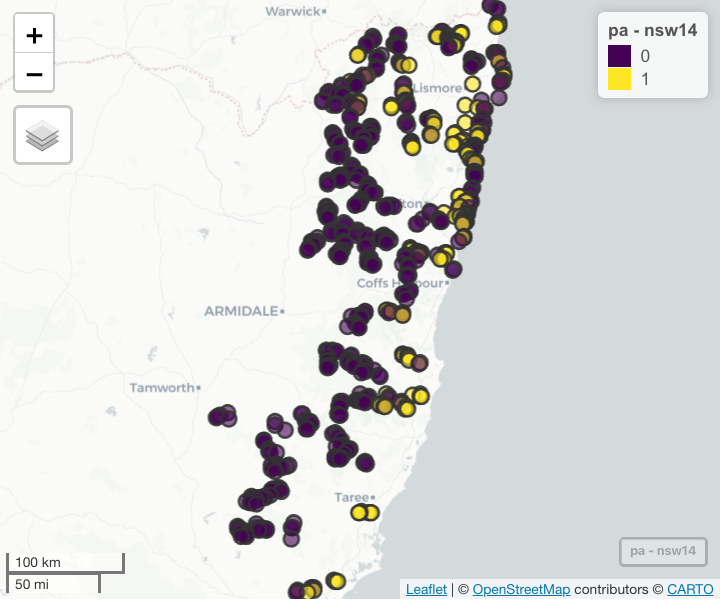Data Visualisation for disdat data

2020-09-09

This document provides example code for understanding and visualising the NCEAS data within the disdat package. This html file is created using R markdown. The code in the grey shaded areas that is not preceded by # can be copied and run in R. The code preceded by ## simply shows what the code above it returns.

We need to load the disdat package first.

# install.packages('disdat')
# devtools::install_github('rspatial/disdat')
library(disdat)

Correlation matrix

Here is one example of how to create correlation matrices showing spearman rank correlation coefficients between all pairs of environmental variables. We use the AWT background data for our sample.

awt <- disBg("AWT")
##   siteid    spid        x       y occ group bc01 bc04 bc05      bc06 bc12 bc15
## 1 100001 pseudo1 423335.8 7888328   0    NA 21.6 1.08 30.2 11.600000 1274  105
## 2 100002 pseudo1 484215.8 7842888   0    NA 23.7 1.06 31.7 12.900000  940  107
## 3 100003 pseudo1 349095.8 8043368   0    NA 18.8 1.02 27.8  9.400001 2177   74
## 4 100004 pseudo1 403655.8 7886248   0    NA 20.5 1.13 29.8 10.300000 1194  102
## 5 100005 pseudo1 366695.8 7970888   0    NA 21.2 1.05 30.1 11.100000 1341   93
## 6 100006 pseudo1 474055.8 7860328   0    NA 22.9 1.04 30.7 12.900000 1059  109
##   bc17 bc20 bc31 bc33      slope topo       tri
## 1   56 19.5   55 0.16 40.7042000   29 540.28050
## 2   41 19.8   76 0.09  0.5810088    3  21.67948
## 3  170 18.5   18 0.65 19.6593600   26 776.79150
## 4   58 19.5   52 0.18  0.0000000    0 138.15930
## 5   87 19.4   44 0.27  9.7593110   22 622.67810
## 6   45 19.7   69 0.11 12.9008700    0 771.08560

Now we can create the correlation matrix for this dataset.

library(GGally)

ggcorr(awt[, 7:ncol(awt)],
method = c("pairwise", "spearman"),
label = TRUE,
label_size = 3,
label_color = "white",
digits = 2) +
theme(legend.justification = c(1, 0),
legend.position = c(0.5, 0.7),
legend.direction = "horizontal") +
guides(fill = guide_colorbar(barwidth = 9,
barheight = 1,
title.position = "top",
title.hjust = 0.5,
title = "Spearman correlation"))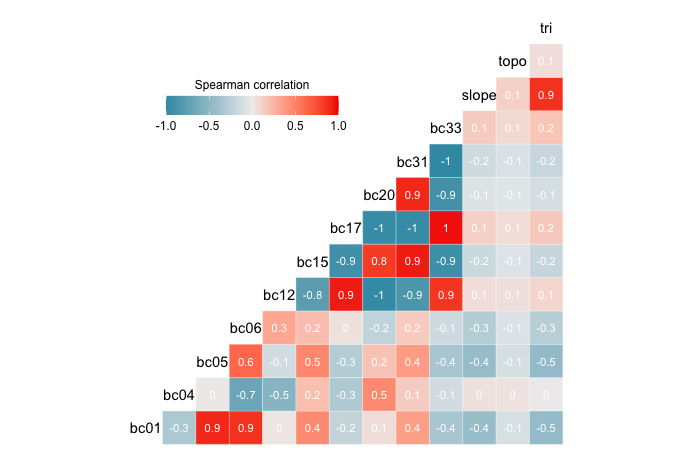Density plots

This code shows how to create a density plot showing the density of records for species (one or many) vs that in the landscape, across one environmental variable in one region. We use all species in AWT across as an example. We plot density of records across variable “bc01”, which is Annual mean temperature.

library(ggplot2)
# create density plot for species presence-only vs background data
# first prepare the species records in the right format for the tidyverse package:
po <- disPo("AWT") # presence-only data
bg <- disBg("AWT") # background data
spdata <- rbind(po, bg)
spdata$occ <- as.factor(spdata$occ)
levels(spdata$occ) <- c("Landscape", "Species") levels(spdata$occ)
##  "Landscape" "Species"
# now plot the data - specify the variable by its column name, as seen below in "aes(x = bc01,.....)"
ggplot(data = spdata, aes(x = bc01, fill = occ)) +
geom_density(alpha = 0.4) +
xlab("Annual mean temperature") +
scale_fill_brewer(palette = "Dark2") +
guides(fill = guide_legend(title = "")) +
theme_bw()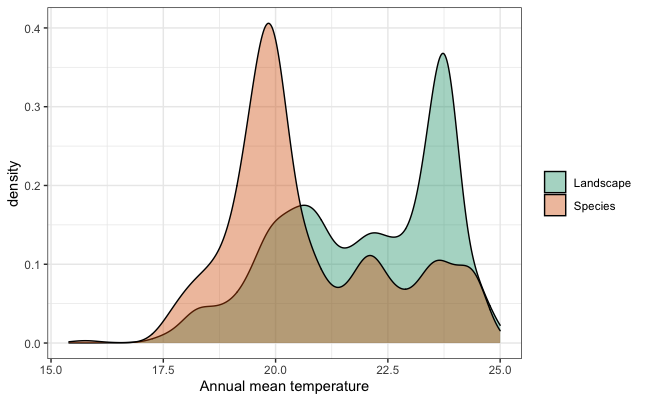Nearest-neighbour distance

You might be interested in distances between sites. There are various reasons for thinking about this, and various ways to calculate it. Here is one example, which simply asks: what is the average nearest-neighbour distance per species in each region, in the PO data. The distance is calculated in metres. This gives an indication of the clustering of sites in a region, which will be influenced by several things: density of sampling, biases in sampling (e.g. do recorders tend to go to the same general areas within the region), whether the selected species are spread throughout the region or clustered.

Example code for calculating the mean nearest-neighbor distance for one region - here, we use AWT:

library(sf)
library(tidyverse)

# presence-only data
awt_sf <- st_as_sf(disPo("AWT"), coords = c("x", "y"), crs = 28355)

# a function to calculate the min distance (excluding self-distance)
mindist <- function(x) {
mindis <- vector(mode = "numeric", length = nrow(x))
for(i in 1:nrow(x)){
mindis[i] <- min(x[i, -i])
}
return(mindis)
}

awt_min_dist <- awt_sf %>%
group_by(spid) %>%
nest() %>%
mutate(distM = map(data, ~st_distance(x = .)),
minDist = map(distM, ~mindist(x = .)),
meanDist = map_dbl(minDist, ~mean(x = .)))

The code produces a “tibble”. You could, for instance, summarise the meanDist column: summary(awt_min_dist$meanDist). If you ran this code for all regions you could then create a figure like this: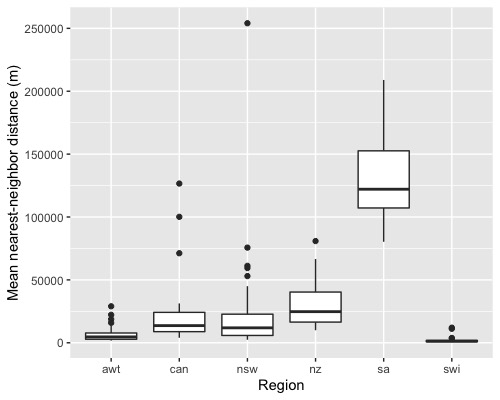Mapping species data This code shows how to map the species presence-only and the evaluation presence-absence data. For doing this we use tmap and mapview packages. Plotting static map We use tmap package to plot species data on one of the polygon borders. library(disdat) library(sf) library(tmap) library(grid) # loading the data r <- disBorder("NSW") # a polygon file showing border of the region podata <- disPo("NSW") # presence-only data padata <- disPa("NSW", "db") # presence-absence of group db for species 'nsw14' # select the species to plot species <- "nsw14" # convert the data.frame to sf objects for plotting po <- st_as_sf(podata[podata$spid == species, ], coords = c("x", "y"), crs = 4326) # subset the species
pa <- st_as_sf(padata, coords = c("x", "y"), crs = 4326)

# create map showing training data
train_sample <- tm_shape(r) + # create tmap object
tm_polygons() + # add the border
tm_shape(po) + # create tmap object for the points to overlay
tm_dots(size = 0.2, col = "blue", alpha = 0.6, legend.show = FALSE) + # add the points
tm_compass(type = "8star", position = c("left", "top")) + # add north arrow
tm_layout(main.title = "Training data", main.title.position = "center") + # manage the layout
tm_grid(lwd = 0.2, labels.inside.frame = FALSE, alpha = 0.4, projection = 4326, # add the grid
labels.format = list(big.mark = ",", fun = function(x){paste0(x, "º")})) # add degree symbol

# create map showing testing data
test_sample <- tm_shape(r) +
tm_polygons() +
tm_shape(pa) +
tm_dots(species, size = 0.2, palette = c("red", "blue"), title = "Occurrence", alpha = 0.6) +
tm_layout(main.title = "Testing data", main.title.position = "center") +
tm_grid(lwd = 0.2, labels.inside.frame = FALSE, alpha = 0.4, projection = 4326,
labels.format = list(big.mark = ",", fun = function(x){paste0(x, "º")}))

# create a layout for putting the maps side-by-side
grid.newpage()
pushViewport(viewport(layout=grid.layout(1,2)))
print(train_sample, vp=viewport(layout.pos.col = 1))
print(test_sample, vp=viewport(layout.pos.col = 2))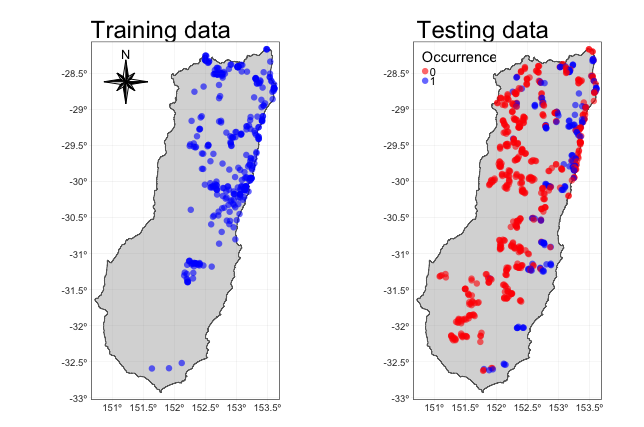We also provided code for creating a whole mapbook of all species data (training presences, and testing presence-absence) – see the disMapBook function.

Plotting interactive map

To have a quick look at the data on an interactive session with satellite imagery background, you can use the sf object created in the previous section in a mapview function. You should be connected to the internet to see the background map. In the map below you should be able to zoom in and out and add different types of background information.

library(mapview)
library(sf)

# get presence-absence data
padata <- disPa(region = "NSW", group = "db")
# use disCRS for each region for its crs code (EPSG)
disCRS("NSW", format = "EPSG")
# convert the data.frame to sf objects for plotting
pa <- st_as_sf(padata, coords = c("x", "y"), crs = disCRS("NSW"))
# select a species to plot
species <- "nsw14"

# plot presence-absence data
# you can add: map.types = "Esri.WorldImagery"
mapview(pa, zcol = species)